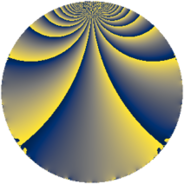# Properties

 Label 175.3.mLevel $175$ Weight $3$ Character orbit 175.m Rep. character $\chi_{175}(34,\cdot)$ Character field $\Q(\zeta_{10})$ Dimension $152$ Newform subspaces $1$ Sturm bound $60$ Trace bound $0$

# Related objects

## Defining parameters

 Level: $$N$$ $$=$$ $$175 = 5^{2} \cdot 7$$ Weight: $$k$$ $$=$$ $$3$$ Character orbit: $$[\chi]$$ $$=$$ 175.m (of order $$10$$ and degree $$4$$) Character conductor: $$\operatorname{cond}(\chi)$$ $$=$$ $$175$$ Character field: $$\Q(\zeta_{10})$$ Newform subspaces: $$1$$ Sturm bound: $$60$$ Trace bound: $$0$$

## Dimensions

The following table gives the dimensions of various subspaces of $$M_{3}(175, [\chi])$$.

Total New Old
Modular forms 168 168 0
Cusp forms 152 152 0
Eisenstein series 16 16 0

## Trace form

 $$152 q - 10 q^{2} + 66 q^{4} - 40 q^{8} - 108 q^{9} + O(q^{10})$$ $$152 q - 10 q^{2} + 66 q^{4} - 40 q^{8} - 108 q^{9} + 24 q^{11} - 21 q^{14} + 60 q^{15} - 150 q^{16} - 50 q^{22} - 180 q^{23} + 20 q^{25} - 45 q^{28} + 24 q^{29} + 170 q^{30} - 155 q^{35} + 136 q^{36} - 10 q^{37} + 250 q^{39} - 305 q^{42} + 202 q^{44} - 342 q^{46} - 178 q^{49} + 260 q^{50} - 220 q^{51} - 60 q^{53} + 78 q^{56} + 50 q^{58} + 100 q^{60} + 190 q^{63} + 424 q^{64} - 220 q^{65} + 710 q^{67} + 270 q^{70} - 236 q^{71} + 690 q^{72} + 28 q^{74} + 360 q^{77} + 80 q^{78} + 374 q^{79} - 688 q^{81} - 370 q^{84} + 490 q^{85} - 522 q^{86} - 2620 q^{88} - 170 q^{91} + 440 q^{92} - 880 q^{95} + 65 q^{98} - 36 q^{99} + O(q^{100})$$

## Decomposition of $$S_{3}^{\mathrm{new}}(175, [\chi])$$ into newform subspaces

Label Dim $A$ Field CM Traces $q$-expansion
$a_{2}$ $a_{3}$ $a_{5}$ $a_{7}$
175.3.m.a $152$ $4.768$ None $$-10$$ $$0$$ $$0$$ $$0$$